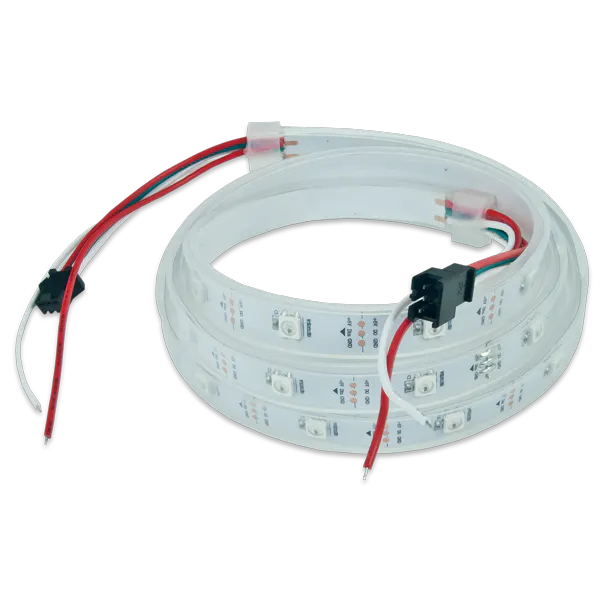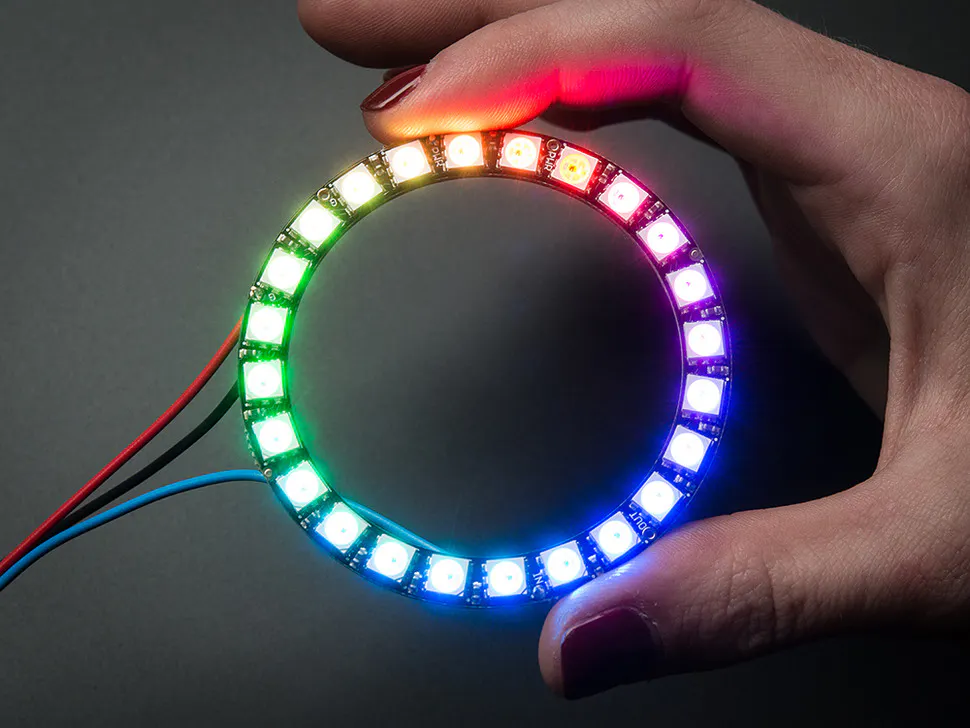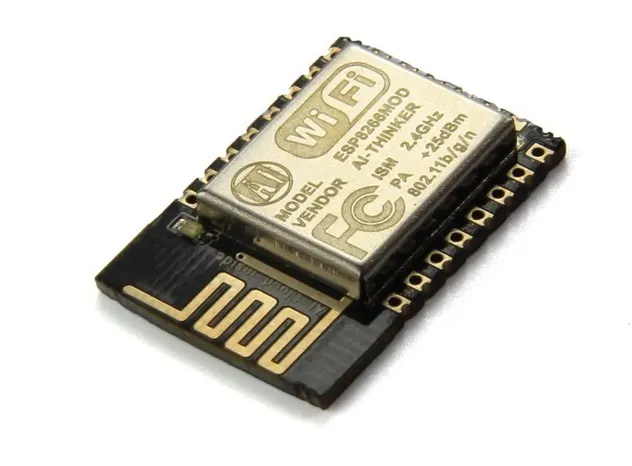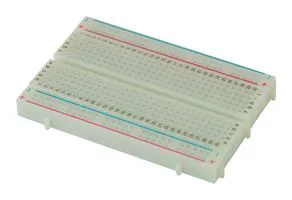RGB 7 Segment display using Neo pixel led

RGB and addressable led, can be supplied with 3.3volts. Using these big panels we can make clock and other display devices.

IntermediateFull instructions provided2 hours6

Things used in this project

Hardware components×1Adafruit NeoPixel Ring: WS2812 5050 RGB LED
×1Espressif ESP8266 ESP-12E
×1

Software apps and online servicesJLCPCB EasyEDA

Hand tools and fabrication machinesSoldering iron (generic)10 Pc. Jumper Wire Kit, 5 cm Long

Custom parts and enclosures

For SMT assembly

CPL file

PICK and Place files for SMT

circuit diagram

Code

Test code for animations and numbers

C/C++

#define PIXELS_PER_SEGMENT  2     // Number of LEDs in each Segment
#define PIXELS_DIGITS       4     // Number of connected Digits
#define PIXELS_PIN          2     // GPIO Pin

Adafruit_NeoPixel strip = Adafruit_NeoPixel(PIXELS_PER_SEGMENT * 7 * PIXELS_DIGITS, PIXELS_PIN, NEO_GRB + NEO_KHZ800);

//Pixel Arrangement
/*
a
f   b
g
e   c
d
*/

// Segment array
byte segments = {
//abcdefg
0b0000001,     // Segment g
0b0000100,     // Segment e
0b0001000,     // Segment d
0b0010000,     // Segment c
0b0100000,     // Segment b
0b1000000,     // Segment a
0b0000010     // Segment f
};

//Digits array
byte digits = {
//abcdefg
0b1111110,     // 0
0b0110000,     // 1
0b1101101,     // 2
0b1111001,     // 3
0b0110011,     // 4
0b1011011,     // 5
0b1011111,     // 6
0b1110000,     // 7
0b1111111,     // 8
0b1110011      // 9
};

//Clear all the Pixels
void clearDisplay() {
for (int i = 0; i < strip.numPixels(); i++) {
strip.setPixelColor(i, strip.Color(0, 0, 0));
}
strip.show();
}

void setup() {
strip.begin();
}

void loop() {
//disp_Seg(200);                // Cycle through all segments        (DelayTime)
disp_Digits(1000);            // Show digits from 0-9              (DelayTime)
//disp_Animation();             // Show some Animations with the segments
//disp_CountUP(500, 450);       // Count numbers in Ascending order  (NUMBER, DelayTime)
//  disp_CountDOWN(500, 250);     // Count numbers in Descending order (NUMBER, DelayTime)
}

void disp_Seg(int wait) {
clearDisplay();
for (int d = 0; d < 5; d++) {
for (int i = 6; i > 0; i--) {
for (int n = 0; n < PIXELS_DIGITS; n++) {
writeSegment(n, i);
}
strip.show();
delay(wait);
}
}
}

void disp_Digits(int wait) {
clearDisplay();
for (int i = 0; i < 10; i++) {
for (int n = 0; n < PIXELS_DIGITS; n++) {
writeDigit(n, i);
}
strip.show();
delay(wait);
}
}

void disp_CountUP(int num, int wait) {
clearDisplay();
for (int i = 0; i <= num; i++) {
writeDigit(0, (i / 100) % 10);
writeDigit(1, (i / 10) % 10);
writeDigit(2, (i / 1) % 10);
strip.show();
delay(wait);
}
}

void disp_CountDOWN(int num, int wait) {
clearDisplay();
for (int i = num; i >= 0; i--) {
writeDigit(0, (i / 100) % 10);
writeDigit(1, (i / 10) % 10);
writeDigit(2, (i / 1) % 10);
strip.show();
delay(wait);
}
}

void disp_Animation() {
clearDisplay();
//UP-DOWN
for (int i = 0; i < 7; i++) {
for (int n = 0; n < PIXELS_DIGITS; n++) writeSegment(n, 5);
strip.show();
delay(100);
for (int n = 0; n < PIXELS_DIGITS; n++) writeSegment(n, 0);
strip.show();
delay(100);
for (int n = 0; n < PIXELS_DIGITS; n++) writeSegment(n, 2);
strip.show();
delay(100);
for (int n = 0; n < PIXELS_DIGITS; n++) writeSegment(n, 0);
strip.show();
delay(100);
for (int n = 0; n < PIXELS_DIGITS; n++) writeSegment(n, 5);
strip.show();
delay(100);
}
//LEFT-RIGHT
for (int i = 0; i < 5; i++) {
for (int n = 0; n < PIXELS_DIGITS; n++) {
writeSegment(n, 6);
strip.show();
delay(150);
}
for (int n = PIXELS_DIGITS - 1; n >= 0; n--) {
writeSegment(n, 3);
strip.show();
delay(150);
}
clearDisplay();
for (int n = 0; n < PIXELS_DIGITS; n++) {
writeSegment(n, 1);
strip.show();
delay(150);
}
for (int n = PIXELS_DIGITS - 1; n >= 0; n--) {
writeSegment(n, 4);
strip.show();
delay(150);
}
clearDisplay();
}
//ZIG-ZAG
for (int i = 0; i < 5; i++) {
for (int n = 0; n < PIXELS_DIGITS; n++) {
writeSegment(n, 6);
strip.show();
delay(125);
clearDisplay();
writeSegment(n, 1);
strip.show();
delay(125);
clearDisplay();
writeSegment(n, 4);
strip.show();
delay(125);
clearDisplay();
writeSegment(n, 3);
strip.show();
delay(125);
clearDisplay();
}
}
}

void writeDigit(int index, int val) {
byte digit = digits[val];
for (int i = 6; i >= 0; i--) {
int offset = index * (PIXELS_PER_SEGMENT * 7) + i * PIXELS_PER_SEGMENT;
uint32_t color;
if (digit & 0x01 != 0) {
if (val == 1) color = strip.Color(50, 0,  0);
if (val == 2) color = strip.Color(50, 50, 0);
if (val == 3) color = strip.Color(50, 0, 50);
if (val == 4) color = strip.Color(0, 50,  0);
if (val == 5) color = strip.Color(0, 50, 50);
if (val == 6) color = strip.Color(0,  0, 50);
if (val == 7) color = strip.Color(50, 25, 0);
if (val == 8) color = strip.Color(25, 5, 75);
if (val == 9) color = strip.Color(75, 25, 5);
if (val == 0) color = strip.Color(5, 75, 25);
}
else
color = strip.Color(0, 0, 0);

for (int j = offset; j < offset + PIXELS_PER_SEGMENT; j++) {
strip.setPixelColor(j, color);
}
digit = digit >> 1;
}
}

void writeSegment(int index, int val) {
byte seg = segments[val];
for (int i = 6; i >= 0; i--) {
int offset = index * (PIXELS_PER_SEGMENT * 7) + i * PIXELS_PER_SEGMENT;
uint32_t color;
if (seg & 0x01 != 0) {
if (val == 0) color = strip.Color(50, 0, 0);
if (val == 1) color = strip.Color(0, 50, 50);
if (val == 2) color = strip.Color(0, 50, 0);
if (val == 3) color = strip.Color(50, 0, 50);
if (val == 4) color = strip.Color(50, 50, 50);
if (val == 5) color = strip.Color(0, 0, 50);
if (val == 6) color = strip.Color(50, 50, 0);
}
else
color = strip.Color(0, 0, 0);

for (int j = offset; j < offset + PIXELS_PER_SEGMENT; j++) {
strip.setPixelColor(j, color);
}
seg = seg >> 1;
}
}

Credits

sagar saini

10 projects • 3 followers
I am sagar saini. A PCB engineer in ISRO and works for national agencies.
Thanks to Electro point.